### IMO Shortlist 1996 problem N3

Kvaliteta:
Avg: 0,0
Težina:
Avg: 7,0
A finite sequence of integers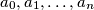$a_0, a_1, \ldots, a_n$ is called quadratic if for each$i$ in the set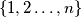$\{1,2 \ldots, n\}$ we have the equality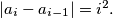$|a_i - a_{i-1}| = i^2.$

a.) Prove that any two integers$b$ and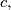$c,$ there exists a natural number$n$ and a quadratic sequence with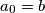$a_0 = b$ and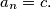$a_n = c.$

b.) Find the smallest natural number$n$ for which there exists a quadratic sequence with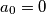$a_0 = 0$ and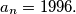$a_n = 1996.$
Izvor: Međunarodna matematička olimpijada, shortlist 1996